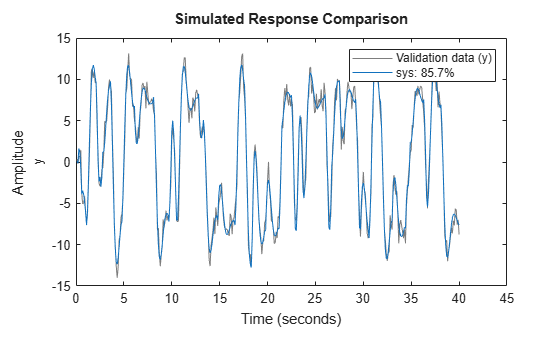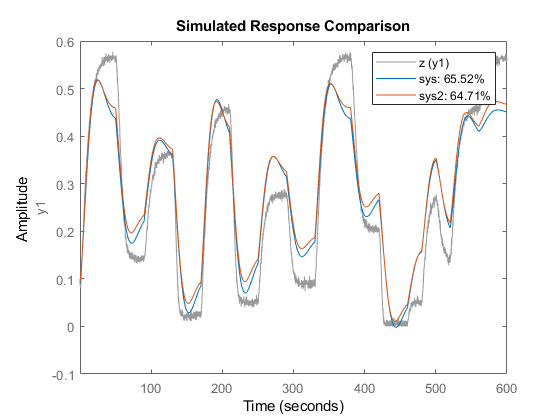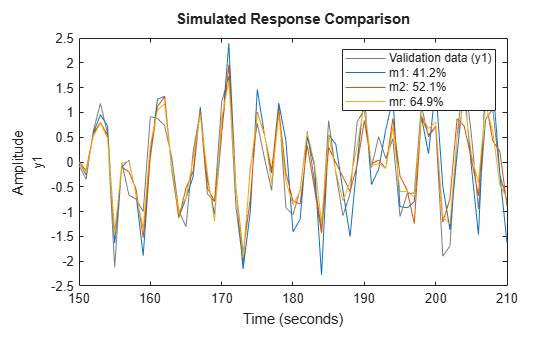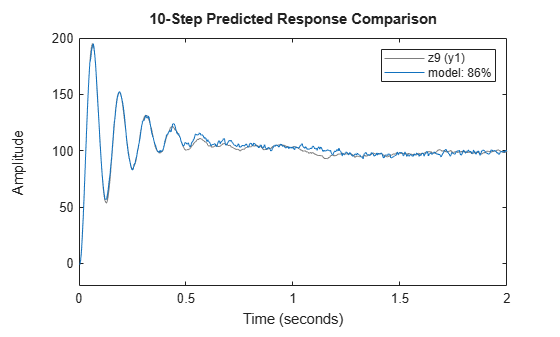armax

Estimate parameters of ARMAX, ARIMAX, ARMA, or ARIMA model using time-domain data

Description

Estimate an ARMAX Model

example

sys = armax(data,[na nb nc nk]) estimates the parameters of an ARMAX or an ARMA idpoly model sys using the prediction-error method and the polynomial orders specified in [na nb nc nk]. The model properties include estimation covariances (parameter uncertainties) and goodness of fit between the estimated and the measured data.

example

sys = armax(data,[na nb nc nk],Name,Value) specifies additional options using one or more name-value pair arguments. For instance, using the name-value pair argument 'IntegrateNoise',1 estimates an ARIMAX or ARIMA model, which is useful for systems with nonstationary disturbances.

Configure Initial Parameters

example

sys = armax(data,init_sys) uses the discrete-time linear model init_sys to configure the initial parameterization.

example

sys = armax(data,___,opt) incorporates an option set opt that specifies options such as estimation objective, handling of initial conditions, regularization, and numerical search method to use for estimation. Specify opt after any of the previous input-argument combinations.

Return Estimated Initial Conditions

example

[sys,ic] = armax(___) returns the estimated initial conditions as an initialCondition object. Use this syntax if you plan to simulate or predict the model response using the same estimation input data and then compare the response with the same estimation output data. Incorporating the initial conditions yields a better match during the first part of the simulation.

Examples

collapse all

Estimate an ARMAX model and view the fit of the model output to the estimation data.

Load the measurement data in iddata object z2.

Estimate an ARMAX model with second-order $\mathit{A}$,$\mathit{B}$, and $\mathit{C}$ polynomials and a transport delay of one sample period.

na = 2;
nb = 2;
nc = 2;
nk = 1;
sys = armax(z2,[na nb nc nk])
sys =
Discrete-time ARMAX model: A(z)y(t) = B(z)u(t) + C(z)e(t)
A(z) = 1 - 1.512 z^-1 + 0.7006 z^-2

B(z) = -0.2606 z^-1 + 1.664 z^-2

C(z) = 1 - 1.604 z^-1 + 0.7504 z^-2

Sample time: 0.1 seconds

Parameterization:
Polynomial orders:   na=2   nb=2   nc=2   nk=1
Number of free coefficients: 6
Use "polydata", "getpvec", "getcov" for parameters and their uncertainties.

Status:
Estimated using ARMAX on time domain data "z2".
Fit to estimation data: 85.89% (prediction focus)
FPE: 1.086, MSE: 1.054

The output displays the polynomial containing the estimated parameters alongside the estimation details. Under Status, Fit to estimation data shows that the estimated model has 1-step-ahead prediction accuracy above 80%.

Compare the model simulated output to the measured data.

compare(z2,sys)The fit of the simulated model to the measured data is nearly the same as the estimation fit.

Estimate an ARMA model and compare its response with both the measured output and an AR model.

Load the data, which contains the time series z9 with noise.

Estimate a fourth-order ARMA model with a first-order $\mathit{C}\text{\hspace{0.17em}}$polynomial.

na = 4;
nc = 1;
sys = armax(z9,[na nc]);

Estimate a fourth-order AR model.

sys_ar = ar(z9,na);

Compare the model outputs with the measured data.

compare(z9,sys,sys_ar)The ARMA model has the better fit to the data.

Estimate an ARMAX model from measured data and specify estimation options.

Load the data and create an iddata object. Initialize option set opt, and set options for Focus, SearchMethod, MaxIterations, and Display. Then estimate the ARMAX model using the updated option set.

z = iddata(y,u,0.2);
opt = armaxOptions;
opt.Focus = 'simulation';
opt.SearchMethod = 'lm';
opt.SearchOptions.MaxIterations = 10;
opt.Display = 'on';
sys = armax(z,[2 2 2 1],opt);

The termination conditions for measured component of the model shown in the progress viewer is that the maximum number of iterations were reached.

To improve results, re-estimate the model using a greater value for MaxIterations, or continue iterations on the previously estimated model as follows:

sys2 = armax(z,sys);
compare(z,sys,sys2)where sys2 refines the parameters of sys to improve the fit to data.

Estimate a regularized ARMAX model by converting a regularized ARX model.

Estimate an unregularized ARMAX model of order 30.

m1 = armax(m0simdata(1:150),[30 30 30 1]);

Estimate a regularized ARMAX model by determining the Lambda value by trial and error.

opt = armaxOptions;
opt.Regularization.Lambda = 1;
m2 = armax(m0simdata(1:150),[30 30 30 1],opt);

Obtain a lower order ARMAX model by converting a regularized ARX model and then performing order reduction.

opt1 = arxOptions;
[L,R] = arxRegul(m0simdata(1:150),[30 30 1]);
opt1.Regularization.Lambda = L;
opt1.Regularization.R = R;
m0 = arx(m0simdata(1:150),[30 30 1],opt1);
mr = idpoly(balred(idss(m0),7));

Compare the model outputs against the data.

opt2 = compareOptions('InitialCondition','z');
compare(m0simdata(150:end),m1,m2,mr,opt2);Estimate a fourth-order ARIMA model for univariate time-series data.

Load data that contains a time series with noise.

Integrate the output signal and use the result to replace the original output signal in z9.

z9.y = cumsum(z9.y);

Estimate a fourth-order ARIMA model with a first-order$\mathit{C}$polynomial by setting 'IntegrateNoise' to true.

model = armax(z9,[4 1],'IntegrateNoise',true);

Predict the model output using 10-step ahead prediction, and compare the predicted output with the estimation data.

compare(z9,model,10)Estimate ARMAX models of varying orders iteratively from measured data.

Load dryer2 data and perform estimation for combinations of polynomial orders na, nb, nc, and input delay nk.

z = iddata(y2,u2,0.08,'Tstart',0);
na = 2:4;
nc = 1:2;
nk = 0:2;
models = cell(1,18);
ct = 1;
for i = 1:3
na_ = na(i);
nb_ = na_;
for j = 1:2
nc_ = nc(j);
for k = 1:3
nk_ = nk(k);
models{ct} = armax(z,[na_ nb_ nc_ nk_]);
ct = ct+1;
end
end
end

Stack the estimated models and compare their simulated responses to the estimation data z.

models = stack(1,models{:});
compare(z,models)Estimate a state-space model of order 3 from the estimation data.

sys0 = n4sid(z2,3);

Estimate an ARMAX model using the previously estimated state-space model to initialize the parameters.

sys = armax(z2,sys0);

Estimate a second-order ARMAX model sys and return the initial conditions in ic.

na = 2;
nb = 2;
nc = 2;
nk = 1;
[sys,ic] = armax(z1i,[na nb nc nk]);
ic
ic =
initialCondition with properties:

A: [2x2 double]
X0: [2x1 double]
C: [0 1]
Ts: 0.1000

ic is an initialCondition object that encapsulates the free response of sys, in state-space form, to the initial state vector in X0. You can incorporate ic when you simulate sys with the z1i input signal and compare the response with the z1i output signal.

Input Arguments

collapse all

Time-domain estimation data, specified as an iddata object. For ARMA and ARIMA time-series models, the input channel in data must be empty. For examples, see ARMA Model and ARIMA Model.

Polynomial orders and delays for the model, specified as a 1-by-4 vector or vector of matrices [na nb nc nk]. The polynomial order is equal to the number of coefficients to estimate in that polynomial.

For an ARMA or ARIMA time-series model, which has no input, set [na nb nc nk] to [na nc]. For an example, see ARMA Model.

For a model with Ny outputs and Nu inputs:

• na is the order of the polynomial A(q), specified as an Ny-by-Ny matrix of nonnegative integers.

• nb is the order of the polynomial B(q) + 1, specified as an Ny-by-Nu matrix of nonnegative integers.

• nc is the order of the polynomial C(q), specified as a column vector of nonnegative integers of length Ny.

• nk is the input-output delay, also known at the transport delay, specified as an Ny-by-Nu matrix of nonnegative integers. nk is represented in ARMAX models by fixed leading zeros of the B polynomial.

For an example, see Estimate ARMAX Model.

System for configuring the initial parameterization of sys, specified as a discrete-time linear model. You obtain init_sys by either performing an estimation using measured data or by direct construction using commands such as idpoly and idss.

If init_sys is an ARMAX model, armax uses the parameter values of init_sys as the initial guess for estimation. To configure initial guesses and constraints for A(q), B(q), and C(q), use the Structure property of init_sys. For example:

• To specify an initial guess for the A(q) term of init_sys, set init_sys.Structure.A.Value as the initial guess.

• To specify constraints for the B(q) term of init_sys:

• Set init_sys.Structure.B.Minimum to the minimum B(q) coefficient values.

• Set init_sys.Structure.B.Maximum to the maximum B(q) coefficient values.

• Set init_sys.Structure.B.Free to indicate which B(q) coefficients are free for estimation.

If init_sys is not a polynomial model with the ARMAX structure, the software first converts init_sys to an ARMAX model. armax uses the parameters of the resulting model as the initial guess for estimating sys.

If opt is not specified and init_sys was obtained by estimation, then the estimation options from init_sys.Report.OptionsUsed are used.

For an example, see Initialize ARMAX Model Parameters Using State-Space Model.

Estimation options for ARMAX model identification, specified as an armaxOptions option set. Options specified by opt include the following:

• Initial condition handling — Use this option to determine how the initial conditions are set or estimated.

• Input and output data offsets — Use these options to remove offsets from data during estimation.

• Regularization — Use this option to control the tradeoff between bias and variance errors during the estimation process.

Name-Value Arguments

Specify optional comma-separated pairs of Name,Value arguments. Name is the argument name and Value is the corresponding value. Name must appear inside quotes. You can specify several name and value pair arguments in any order as Name1,Value1,...,NameN,ValueN.

Example: 'InputDelay',2 applies an input delay of two sample periods to all input channels

Input delays expressed as integer multiples of the sample time, specified as the comma-separated pair consisting of 'InputDelay' and one of the following:

• Nu-by-1 vector, where Nu is the number of inputs — Each entry is a numerical value representing the input delay for the corresponding input channel.

• Scalar value — Apply the same delay to all input channels.

• 0 — No input delays.

Example: armax(data,[2 1 1 0],'InputDelay',1) estimates a second-order ARX model with first-order B and C polynomials that has an input delay of two samples.

Transport delays for each input-output pair, expressed as integer multiples of the sample time, and specified as the comma-separated pair consisting of 'IODelay' and one of the following:

• Ny-by-Nu matrix, where Ny is the number of outputs and Nu is the number of inputs — Each entry is an integer value representing the transport delay for the corresponding input-output pair.

• Scalar value — Apply the same delay to all input-output pairs.

'IODelay' is useful as a replacement for the nk order. You can factor out max(nk-1,0) lags as the 'IODelay' value. For nk > 1, armax(na,nb,nk) is equivalent to armax(na,nb,1,'IODelay',nk-1).

Addition of integrators in the noise channel, specified as the comma-separated pair consisting of 'IntegrateNoise' and a logical vector of length Ny, where Ny is the number of outputs.

Setting 'IntegrateNoise' to true for a particular output results in the model

$A\left(q\right)y\left(t\right)=B\left(q\right)u\left(t-nk\right)+\frac{C\left(q\right)}{1-{q}^{-1}}e\left(t\right)$

where $\frac{1}{1-{q}^{-1}}$ is the integrator in the noise channel, e(t).

Use 'IntegrateNoise' to create ARIMA or ARIMAX models.

For an example, see ARIMA Model.

Output Arguments

collapse all

ARMAX model that fits the given estimation data, returned as a discrete-time idpoly object. This model is created using the specified model orders, delays, and estimation options.

Information about the estimation results and options used is stored in the Report property of the model. Report has the following fields.

Report FieldDescription
Status

Summary of the model status, which indicates whether the model was created by construction or obtained by estimation.

Method

Estimation command used.

InitialCondition

Handling of initial conditions during model estimation, returned as one of the following values:

• 'zero' — The initial conditions were set to zero.

• 'estimate' — The initial conditions were treated as independent estimation parameters.

• 'backcast' — The initial conditions were estimated using the best least squares fit.

This field is especially useful to view how the initial conditions were handled when the InitialCondition option in the estimation option set is 'auto'.

Fit

Quantitative assessment of the estimation, returned as a structure. See Loss Function and Model Quality Metrics for more information on these quality metrics. The structure has the following fields:

FieldDescription
FitPercent

Normalized root mean squared error (NRMSE) measure of how well the response of the model fits the estimation data, expressed as the percentage fitpercent = 100(1-NRMSE).

LossFcn

Value of the loss function when the estimation completes.

MSE

Mean squared error (MSE) measure of how well the response of the model fits the estimation data.

FPE

Final prediction error for the model.

AIC

Raw Akaike Information Criteria (AIC) measure of model quality.

AICc

Small-sample-size corrected AIC.

nAIC

Normalized AIC.

BIC

Bayesian Information Criteria (BIC).

Parameters

Estimated values of model parameters.

OptionsUsed

Option set used for estimation. If no custom options were configured, this is a set of default options. See armaxOptions for more information.

RandState

State of the random number stream at the start of estimation. Empty, [], if randomization was not used during estimation. For more information, see rng.

DataUsed

Attributes of the data used for estimation, returned as a structure with the following fields.

FieldDescription
Name

Name of the data set.

Type

Data type.

Length

Number of data samples.

Ts

Sample time.

InterSample

Input intersample behavior, returned as one of the following values:

• 'zoh' — Zero-order hold maintains a piecewise-constant input signal between samples.

• 'foh' — First-order hold maintains a piecewise-linear input signal between samples.

• 'bl' — Band-limited behavior specifies that the continuous-time input signal has zero power above the Nyquist frequency.

InputOffset

Offset removed from time-domain input data during estimation. For nonlinear models, it is [].

OutputOffset

Offset removed from time-domain output data during estimation. For nonlinear models, it is [].

Termination

Termination conditions for the iterative search used for prediction error minimization, returned as a structure with the following fields:

FieldDescription
WhyStop

Reason for terminating the numerical search.

Iterations

Number of search iterations performed by the estimation algorithm.

FirstOrderOptimality

$\infty$-norm of the gradient search vector when the search algorithm terminates.

FcnCount

Number of times the objective function was called.

UpdateNorm

Norm of the gradient search vector in the last iteration. Omitted when the search method is 'lsqnonlin' or 'fmincon'.

LastImprovement

Criterion improvement in the last iteration, expressed as a percentage. Omitted when the search method is 'lsqnonlin' or 'fmincon'.

Algorithm

Algorithm used by 'lsqnonlin' or 'fmincon' search method. Omitted when other search methods are used.

For estimation methods that do not require numerical search optimization, the Termination field is omitted.

Estimated initial conditions, returned as an initialCondition object or an object array of initialCondition values.

• For a single-experiment data set, ic represents, in state-space form, the free response of the transfer function model (A and C matrices) to the estimated initial states (x0).

• For a multiple-experiment data set with Ne experiments, ic is an object array of length Ne that contains one set of initialCondition values for each experiment.

If armax returns ic values of 0 and the you know that you have non-zero initial conditions, set the 'InitialCondition' option in armaxOptions to 'estimate' and pass the updated option set to armax. For example:

opt = armaxOptions('InitialCondition','estimate')
[sys,ic] = armax(data,np,nz,opt)
The default 'auto' setting of 'InitialCondition' uses the 'zero' method when the initial conditions have a negligible effect on the overall estimation-error minimization process. Specifying 'estimate' ensures that the software estimates values for ic.

For more information, see initialCondition. For an example of using this argument, see Obtain Initial Conditions.

collapse all

ARMAX Model

The ARMAX (Autoregressive Moving Average with Extra Input) model structure is:

A more compact way to write the difference equation is

$A\left(q\right)y\left(t\right)=B\left(q\right)u\left(t-{n}_{k}\right)+C\left(q\right)e\left(t\right)$

where

• $y\left(t\right)$ — Output at time $t$

• ${n}_{a}$ — Number of poles

• ${n}_{b}$ — Number of zeroes plus 1

• ${n}_{c}$ — Number of C coefficients

• ${n}_{k}$ — Number of input samples that occur before the input affects the output, also called the dead time in the system

• $y\left(t-1\right)\dots y\left(t-{n}_{a}\right)$ — Previous outputs on which the current output depends

• $u\left(t-{n}_{k}\right)\dots u\left(t-{n}_{k}-{n}_{b}+1\right)$ — Previous and delayed inputs on which the current output depends

• $e\left(t-1\right)\dots e\left(t-{n}_{c}\right)$ — White-noise disturbance value

The parameters na, nb, and nc are the orders of the ARMAX model, and nk is the delay. q is the delay operator. Specifically,

$A\left(q\right)=1+{a}_{1}{q}^{-1}+\dots +{a}_{{n}_{a}}{q}^{-{n}_{a}}$

$B\left(q\right)={b}_{1}+{b}_{2}{q}^{-1}+\dots +{b}_{{n}_{b}}{q}^{-{n}_{b}+1}$

$C\left(q\right)=1+{c}_{1}{q}^{-1}+\dots +{c}_{{n}_{c}}{q}^{-{n}_{c}}$

ARMA Time-Series Model

The ARMA (Autoregressive Moving Average) model is a special case of an ARMAX Model with no input channels. The ARMA single-output model structure is given by the following equation:

$A\left(q\right)y\left(t\right)=C\left(q\right)e\left(t\right)$

ARIMAX Model

The ARIMAX (Autoregressive Integrated Moving Average with Extra Input) model structure is similar to the ARMAX model, except that it contains an integrator in the noise source e(t):

$A\left(q\right)y\left(t\right)=B\left(q\right)u\left(t-nk\right)+\frac{C\left(q\right)}{\left(1-{q}^{-1}\right)}e\left(t\right)$

ARIMA Model

The ARIMA (Autoregressive Integrated Moving Average) model structure is a reduction of the ARIMAX model with no inputs:

$A\left(q\right)y\left(t\right)=\frac{C\left(q\right)}{\left(1-{q}^{-1}\right)}e\left(t\right)$

Algorithms

An iterative search algorithm minimizes a robustified quadratic prediction error criterion. The iterations are terminated when any of the following is true:

• Maximum number of iterations is reached.

• Expected improvement is less than the specified tolerance.

• Lower value of the criterion cannot be found.

You can get information about the stopping criteria using sys.Report.Termination.

Use the armaxOptions option set to create and configure options affecting the estimation results. In particular, set the search algorithm attributes, such as MaxIterations and Tolerance, using the 'SearchOptions' property.

When you do not specify initial parameter values for the iterative search as an initial model, they are constructed in a special four-stage LS-IV algorithm.

The cutoff value for the robustification is based on the Advanced.ErrorThreshold estimation option and on the estimated standard deviation of the residuals from the initial parameter estimate. The cutoff value is not recalculated during the minimization. By default, no robustification is performed; the default value of ErrorThreshold option is 0.

To ensure that only models corresponding to stable predictors are tested, the algorithm performs a stability test of the predictor. Generally, both $C\left(q\right)$ and $F\left(q\right)$ (if applicable) must have all zeros inside the unit circle.

Minimization information is displayed on the screen when the estimation option 'Display' is 'On' or 'Full'. When 'Display' is 'Full', both the current and the previous parameter estimates are displayed in column-vector form, and the parameters are listed in alphabetical order. Also, the values of the criterion function (cost) are given and the Gauss-Newton vector and its norm are displayed. When 'Display' is 'On', only the criterion values are displayed.

Alternatives

armax does not support continuous-time model estimation. Use tfest to estimate a continuous-time transfer function model, or ssest to estimate a continuous-time state-space model.

armax supports only time-domain data. For frequency-domain data, use oe to estimate an Output-Error (OE) model.

 Ljung, L. System Identification: Theory for the User, Second Edition. Upper Saddle River, NJ: Prentice-Hall PTR, 1999. See chapter about computing the estimate.

System Identification Toolbox DocumentationGet trial now